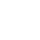CurrentHome>Resources
Reflection Measurement of S3602 Vector Network Analyzer
Release time :2021.03.24 Reading quantity:46

Saluki S3602 series vector network analyzer has multiple calibration modes such as frequency response, single port, response isolation, enhanced response, double ports and electrical calibration, and multiple display modes such as the logarithmic amplitude, linearity amplitude, standing wave, phase, group delay and Smith chart. It is equipped with various standard interfaces, such as USB, LAN, GPIB and VGA.

This section introduces the basic knowledge of reflection measurement.

1. Introduction of Reflection Measurement

Reflection measurement is an important part of network measurement. At first, the reflection measurement will be introduced. In order to better understand reflection measurement, the optical wave is applied to simulate the traveling wave transmission along the transmission line.

If any optical element such as the lens is set, part of light will be reflected by the lens, but the majority of light will be transmitted continuously through the lens. If the surface of the optical element is a mirror, the majority of light will be reflect, and only a little light will be transmitted or no light will be transmitted.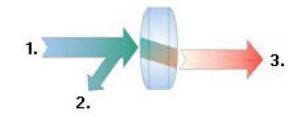Fig.1 Light reflection and transmission

The RF signal will be reflected if the impedance of two connectors is different from each other. Reflection measurement is a process to measure the ratio of the reflected signal and incident signal. The incident signal is measured by Receiver R, and the reflected signal is measured by Receiver A. Therefore, the result of reflection measurement is a ratio of A and R (i.e. A/R). The reflection characteristic of the tested device can be fully quantified with the signal amplitude and phase information of Receiver A and R. Among the S-parameter terms, S11 represents the reflection of Port 1 (input port) of the tested device and S22 represents the reflection of Port 2 (input port) of the tested device. Reflection measurement aims to ensure the efficient transmission of RF energy. If energy is reflected, only a little energy will be transmitted to the expected place. In addition, if too much energy is reflected, the device may be burnt, such as the output power amplifier.

2. Reflection Measurement Expression

The data can be expressed in various methods according to the requirements.

2.1 Return Loss

A simple way to express reflection data is the return loss, which is a scalar in dB. The return loss refers to the dB difference between the reflected signal and incident signal. If the impedance matches exactly, the return loss will be infinite. For the open, short or no-loss reactive circuit, the return loss is 0dB. For example, if the logarithmic format is applied in measurement, the reflection measurement data will be -18dB, indicating that the return loss is 18dB (ignoring the minus sign).

2.2 Standing Wave Ratio

The standing wave will be produced if two groups of waves are transmitted in the opposite directions along one transmission line. In this case, the voltage standing wave ratio (VSWR or SWR for short) can be applied. SWR is defined as the ratio of the maximum RF envelope voltage and minimum RF envelope voltage at the given frequency. It is a scalar. If the impedance matches exactly, SWR is 1. For the open, short or no-loss reactive circuit, SWR is infinite.

2.3 Reflection Coefficient

Another way to express reflection measurement is the reflection coefficient (Γ), including the amplitude and phase information. The amplitude part of Γ is referred to as ρ. The reflection coefficient is a ratio of the reflection voltage and incident voltage. The ρ value range is 0-1. If the transmission line is connected with characteristic impedance, all the energy will be transmitted to the load, with no energy reflection ρ=0. If the transmission line is connected through the open-circuit device or short-circuit device, all the energy will be reflected ρ=1. There is no unit of measurement for ρ.

Below is the phase information of reflection. If the signal wavelength is larger than the conductor length at high frequency, the reflected wave can be regarded as the wave transmitted in the direction opposite to the incident wave. The standing wave will be produced in case of mixing of the incident and reflected wave. In this case, the voltage- enveloped amplitude will change along with the transmission line position.

If the transmission line is connected with characteristic impedance, with no reflection signal, the energy will be transmitted in one direction along the transmission line, and all the energy of the incident signal will be transmitted to the load, as shown in the Fig.2.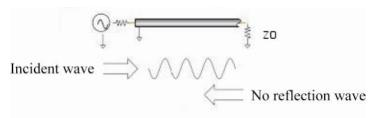Fig.2 Signal transmission of transmission line connected through load

If the transmission line is connected through the short-circuit device, all the energy will be reflected to the signal source, and the amplitude of the reflected wave will be the same as that of the incident wave (ρ=1), and the voltage of both ends of the short-circuit device is 0. The phase of the reflected voltage wave of the short-circuit point is the same as that of the incident voltage wave (180-degree difference), and the voltage will be counterbalanced.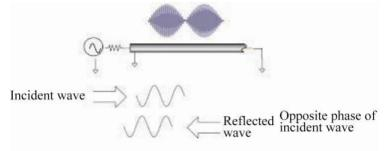Fig.3 Signal transmission of transmission line connected through short-circuit device

If the transmission line is connected through the open-circuit device, all the energy will be reflected to the signal source, the amplitude of the reflected wave is the same as that of the incident wave (ρ=1), no current flows through the open-circuit device, and the reflected voltage wave is the same as the incident voltage wave.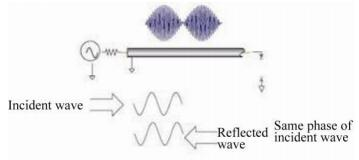Fig.4 Signal transmission of transmission line connected through open-circuit device

If the transmission line is connected through a 25Ω resistor, part of the energy will be absorbed, and part of the energy will be reflected to the signal source. The amplitude of the reflected wave is 1/3 of that of the incident wave. The voltage is subject to 180-degree difference at the resistor. The phase relationship changes with the distance of the end resistor along the transmission line. The valley of the standing wave figure is not approaching zero, and the peak is smaller than that of the open-circuit device and short-circuit device.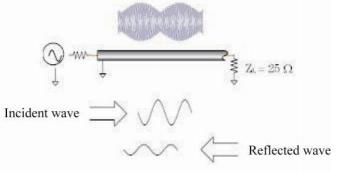Fig.5 Signal transmission of transmission line connected through 25Ω Resistor

2.4 Impedance

The impedance is another way to express the reflection data. The data format refers to the display of measurement data in the graphical form. Select the data format which is the most favorable to understand the characteristics of the tested device.